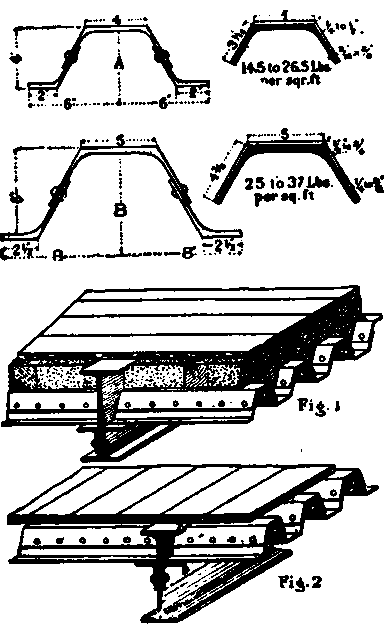## 232. Railings For Roof Protection

In cities, where houses adjoin each other and have their roofs on the same level, the roofs are protected from thieves by wrought-iron picket railings made of 1/2-inch to 3/4-inch diameter bars, from 3 to 5 inches apart between centres and from 5 to 7 feet in height, extending in a quarter circle over front of cornice. The longitudinal bars are 1 1/2 X 1/2-inch flat iron. The pickets are single or double pointed, in fact made as jagged as possible.

## 233. Pipe Railing

For neat, inexpensive and substantial railings, pipes connected and screwed into couplings are the simplest. The rails can be bent to any desired pitch and angle. The standards or posts are also of pipe, and are set into and secured to cast-iron sockets, and further secured to the coping or base upon which they set by expansion bolts.

## 234. Corrugated Flooring

The trough-shaped sections as shown are now successfully used in the floors of buildings as well as bridges. The smaller section, A, is generally applied to buildings as shown at Fig. 1, while the larger section, B, is better adapted for bridges, as Fig. 2.* Taken from Pencoyd Iron Works Handbook.

The following table gives weight per square foot of floor surface for different thicknesses of the section; also the moments of resistance for one foot in width.

 Section. A A A A A B B B B B Weight per square foot in pounds. 14.5 17.5 20.5 23.5 26.5 24.5 27.5 30.5 33.5 36.5 Moment of resistance for 1 foot in width. 4.41 5.50 6.6l 7.74 8.89 11.56 13.06 14.57 16.12 17.67

## 235. Tanks

An ordinary tank, open to the atmosphere, will have no other strain to withstand beyond that which is due to the weight of water in it; hence the maximum strain on the plates will be reached when the tank is full of water. The tank made of wrought-iron plates should, if of any considerable depth, be strengthened at intervals by angles or tee irons, in order to prevent the sides bulging out; and this being the case, the proper thickness of plate at any depth can be found from the following Rule. To find the thickness in inches of any plate necessary to resist the pressure of the water, divide the distance in feet between the strengthening ribs by 20, and multiply the quotient by the square root of the depth in feet from the surface of the water to the plate under consideration.

For example: Let a tank be 10 feet deep, the distance between ribs 3 feet; then the depth of the bottom plates will be 10 feet. Hence, as 3.16 is the square root of 10, we proceed thus:

20)3 feet = distance between ribs.

0.15

3.16 = square root of depth.

90

15 45

.4740 inch = thickness of bottom plate. Or the thickness of bottom plate should be not less than 1/2 inch.

Having found the thickness of bottom plate, the next step is to determine that of the side plates. The thickness must be calculated for the depth of the lower edges of each tier of plates where the maximum strain will come. A tank of the dimensions assumed would be made with three tiers of plates, forming the sides, each tier being 3 feet 4 inches deep, so as to make up a total length of 10 feet of the tank, and upon these depths we may base our calculations.

The bottom tier of plates should be of the same thickness as the bottom plates of the tank, that is 1/2 inch, as their lower edges are the same distance below the surface as the bottom plates. The lower edge of the second or middle tier of plates will be 6 feet 8 inches (6.67 feet) below the top of the tank.

20)3 feet = distance between ribs.

0.15

2.58 = square root of depth in feet.

120

75 30

.3870 inch = thickness of middle tier of plates. The middle tier should therefore be 7/16 inch thick.

The lower edge of the top row of plates will be 3 feet 4 inches (3.34 feet) below the top of tank.

20)3 feet = distance between ribs.

0.15

1.82 = square root of depth.

30 120 15

.2730 inch = the thickness of top row. So the top row should be 5/16 inch thick.

The angle irons, tee irons and cover strips should be proportionate to the thicknesses of the plates. The 1/2-inch plate should be fastened with 3/4-inch rivets, the cover strips 4 inches wide. The angle iron for joining the bottom of tank to the sides should be 2 1/2" X 2 1/2" X 1/2", thus allowing 2 inches width of plate for each rivet, which may be 2 inches pitch. Vertical tee irons should be 4" X 3" X 1/2". The5/16-inch plates should have 5/8-inch rivets, with cover strips 3 1/2 inches wide, and stiffening tee irons 4" X 3" X 1/2" - the 5/16-inch plates to be jointed the same as the 7/16-inch. The vertical stiffeners should be bent across the top of the tank so as to form frames; and the edges of the tank may be stiffened by angle irons 3 X 3 X3/8 inch.